Question of The Day11-12-2019

What is the area of the shaded region if the area of the square is 100 cm2?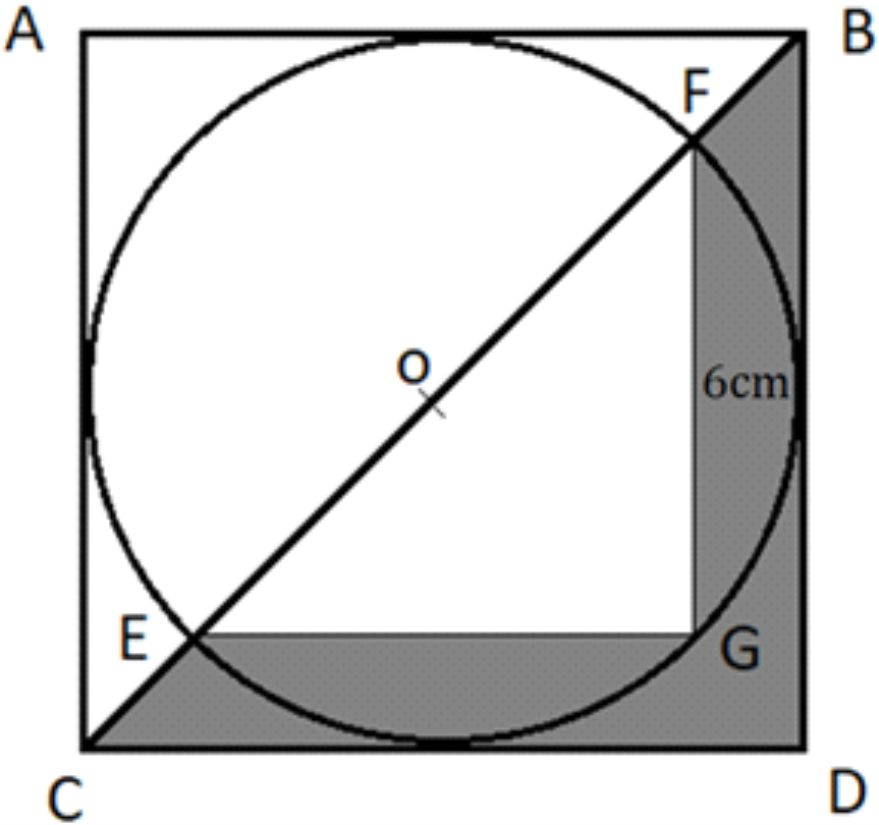Correct Answer : a ) 26 cm2

Explanation :

Considering the given figureGiven:

Area of the square (AS) is = 100 cm2 …… (1)

So,

Side of the square =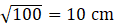Thus, the Diameter of the circle will be 10 cm

Property: Angle subtended by the two ends of diameter with any other point on the circumference of a circle is always 900.

So,

∠EGF = 900

So,

In triangle EGF using Pythagoras theorem

(EF)2 = (EG)2 + (FG)2

EG =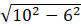EG = 8 cm

Therefore,

Area of the triangle EGF will be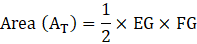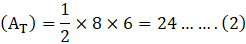So, the area of the shaded region will be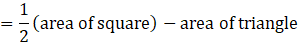From equation (1) and (2) we get

Area of shaded region = 50 – 24

= 26 cm2

Hence, (a) is the correct answer.

Such type of question is asked in different government exams like SSC CGL, SSC MTS, SSC CPO, SSC CHSL, RRB JE, RRB NTPC, RRB GROUP D, RRB OFFICER SCALE-I, IBPS PO, IBPS SO, RRB Office Assistant,IBPS Clerk, RBI Assistant, IBPS RRB OFFICER SCALE 2&3, etc.

Ready and attempt free mock tests at and boost your preparation to excel in the actual exam.0